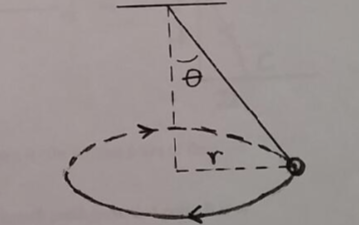# Problem: A ball of mass m = 1.30 kg is attached to a massless rope and swung in a horizontal circle of radius r = 1.70 m, as shown in the figure. The rope makes a constant angle θ with the vertical as the ball swings around the circle, and the ball makes one revolution around the circle in a time Δt = 3.00 s. Calculate the centripetal acceleration of the ball.

87% (49 ratings)
###### Problem Details

A ball of mass m = 1.30 kg is attached to a massless rope and swung in a horizontal circle of radius r = 1.70 m, as shown in the figure. The rope makes a constant angle θ with the vertical as the ball swings around the circle, and the ball makes one revolution around the circle in a time Δt = 3.00 s.

Calculate the centripetal acceleration of the ball.Frequently Asked Questions

What scientific concept do you need to know in order to solve this problem?

Our tutors have indicated that to solve this problem you will need to apply the Centripetal Forces concept. You can view video lessons to learn Centripetal Forces. Or if you need more Centripetal Forces practice, you can also practice Centripetal Forces practice problems.

How long does this problem take to solve?

Our expert Physics tutor, Jeffery took 2 minutes and 10 seconds to solve this problem. You can follow their steps in the video explanation above.